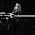# Concept of Mass

### Mass

1. Mass of an object is the amount of matter contained in the object.
2. The SI unit for mass is kilogram (kg). Mass can also be measured in gram (g) and milligram (mg).
1 kg = 1000 g
1 g =1000 mg
3. Mass is constant and not influenced by any gravitational force.
4. Mass is measured using
1. lever balance
2. beam balance
3. double beam balance
4. triple beam balance
5. electronic balance
5. In the laboratory, mass of objects can be measured using a lever balance.

### Weight

1. The weight of an object is the pull of gravity on it.
2. The SI unit for weight is Newton (N).
3. In the laboratory, weight is measured using a spring balance and a compression balance.
4. Weight varies from place to place, depending on the strength of the Earth's gravitational pull.
5. Weight is heavier when measured in North Pole and South Pole than when measured in the equatorial regions due to stronger gravitational pull.
6. When an object moves away from the Earth, its weight decreases as the Earth's gravitational force decreases.

### Differences Between Mass and Weight

The following chart shows the differences between mass and weight:
 Weight Mass Depends on the gravitational field strength Independent from the gravitational field strength Vector quantity Scalar Quantity Unit Newton (N) Unit: Kilogram (kg)

1.2.